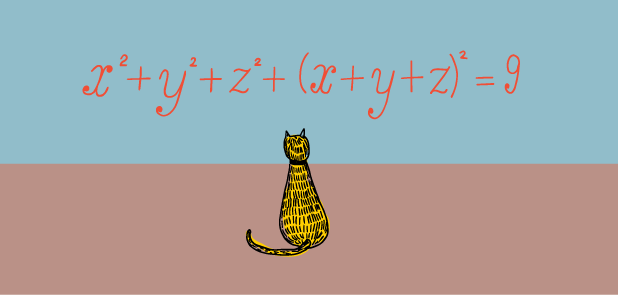# Almost A Sphere, But Not QuiteLet $x,y,z$ be real numbers such that $x^2+y^2+z^2+(x+y+z)^2=9$ and $xyz\leq \frac{15}{32}$. To 2 decimal places, what is the greatest possible value of $x$?

×